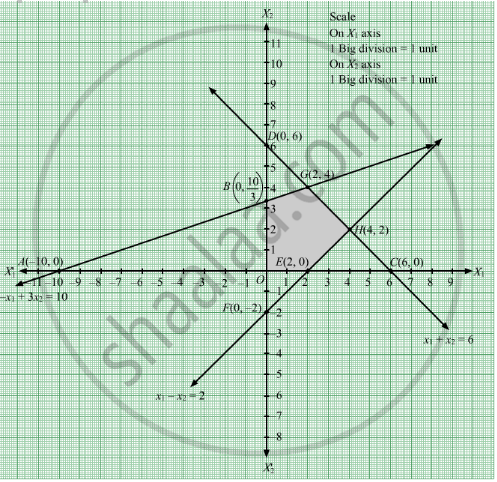# Maximize Z = −X1 + 2x2 Subject to − X 1 + 3 X 2 ≤ 10 X 1 + X 2 ≤ 6 X 1 − X 2 ≤ 2 X 1 , X 2 ≥ 0 - Mathematics

Sum

Maximize Z = −x1 + 2x2
Subject to

$- x_1 + 3 x_2 \leq 10$
$x_1 + x_2 \leq 6$
$x_1 - x_2 \leq 2$
$x_1 , x_2 \geq 0$

#### Solution

First, we will convert the given inequations into equations, we obtain the following equations:
x1 + 3x2 = 10, x1 + x2 = 6, x1 + x2 = 2, x1 = 0 and x2 = 0
Region represented by −x1 + 3x2 ≤ 10:
The line −x1 + 3x2 = 10 meets the coordinate axes at A(10, 0) and $B\left( 0, \frac{10}{3} \right)$ respectively. By joining these points we obtain the line −x1 + 3x2 = 10.
Clearly (0,0) satisfies the inequation −x1 + 3x2 ≤ 10 .So,the region in the plane which contain the origin represents the solution set of the inequation
−x1 + 3x2 ≤ 10.

Region represented by x1 + x2 ≤ 6:
The line x1 + x2 = 6 meets the coordinate axes at C(6, 0) and D(0, 6) respectively. By joining these points we obtain the line x1 + x2 = 6.Clearly (0,0) satisfies the inequation x1 + x2 ≤ 6. So,the region containing the origin represents the solution set of the inequation x1 + x2 ≤ 6.

Region represented by x1 x2 ≤ 2:
The line x1 − x2 = 2 meets the coordinate axes at E(2, 0) and F(0, 2) respectively. By joining these points we obtain the line x1 − x2 = 2.Clearly (0,0) satisfies the inequation x1 x2 ≤ 2. So,the region containing the origin represents the solution set of the inequation x1 x2 ≤ 2.

Region represented by x1 ≥ 0 and x2 ≥ 0:
Since, every point in the first quadrant satisfies these inequations. So, the first quadrant is the region represented by the inequations x1 ≥ 0 and x2 ≥ 0.

The feasible region determined by the system of constraints, −x1 + 3x2 ≤ 10, x1 + x2 ≤ 6, x1 x2 ≤ 2x1 ≥ 0, and x2 ≥ 0, are as follows.The corner points of the feasible region are O(0, 0), E(2, 0), H(4, 2), G(2, 4) and $B\left( 0, \frac{10}{3} \right)$ .

The values of Z at these corner points are as follows.

 Corner point Z = −x1 + 2x2 O(0, 0) −1 × 0 + 2 × 0 = 0 E(2, 0) −1 × 2 + 2 × 0 = −2 H(4, 2) −1 × 4 + 2 × 2 = 0 G(2, 4) −1 × 2 + 2 × 4 = 6 $B\left( 0, \frac{10}{3} \right)$ −1 × 0 + 2 × $\frac{10}{3}$= $\frac{20}{3}$

We see that the maximum value of the objective function Z is $\frac{20}{3}$which is at $B\left( 0, \frac{10}{3} \right)$ .

Concept: Graphical Method of Solving Linear Programming Problems
Is there an error in this question or solution?

#### APPEARS IN

RD Sharma Class 12 Maths
Chapter 30 Linear programming
Exercise 30.2 | Q 18 | Page 33